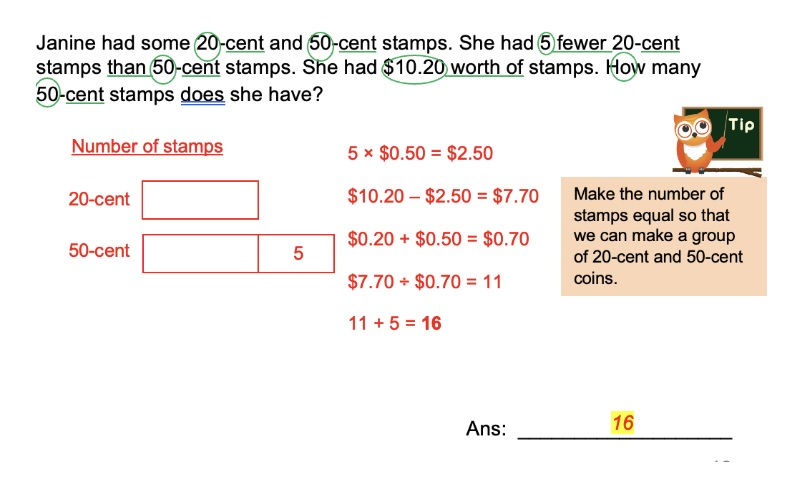top of page
Search

# Stop Losing Marks: 6 Tips to Ace PSLE Maths 2 Marks QuestionsAre you worried about your child's PSLE Math exam? Do you notice them losing marks on seemingly effortless 2-mark questions? These P6 PSLE Math questions require students to not only understand the concepts but also apply them with the right answering technique to score marks.

It's quite common for students to face difficulties with certain questions, but with proper guidance and support, you can empower your child to overcome these challenges and become proficient in Math. In this article, our educator shares six helpful tips to help your child secure 2-mark questions and boost their PSLE Math scores. So take advantage of this opportunity to share it with your child!

## Tips to Ace PSLE Maths 2 Marks Questions### Tip 1: Read the Question Carefully

One crucial tip to ace Primary 6 PSLE Maths 2 Marks questions is to read the question carefully. Rushing through the question may cause you to miss crucial details, such as the units of measurement. Instead, take the time to read and understand what the question is asking for, and feel free to double-check your work before submitting your answer. Remember, attention to detail can make all the difference in your score!

Example:

Max has a tank which measures 5 cm by 14 cm by 28 cm. The tank was filled to the brim at first. Then 250 ml of water leaked from the tank. How much water was left in the tank?

Note: 1 cm³ = 1 ml5 cm x 14 cm x 28 cm = 1960 cm³

250 ml = 250 ml cm³

1960 cm³ - 250 cm³ = 1710 cm³

= 1.71 l

Ans: 171 l

### Tip 2: Identify Keywords or Phrases to Determine the Correct Problem-Solving Approach

Look for keywords or phrases that can help you identify the correct approach to solve the PSLE Math problem. Identifying these keywords will help you understand what the question is asking for and determine the appropriate method to solve the problem. By doing so, you can save time and avoid unnecessary errors.

Example:

There were 84 red beads and 57 blue beads in a container. Wendy added an equal number of blue and red beads to it. The ratio of the number of red beads to the number of blue beads became 11: 8. How many red beads were there in the end?

Tip: Since an equal amount was added, the difference between red and blue beads remains unchanged. Concept: Difference unchanged

Solution:### Tip 3: Show Your WorkingAlways show your work, even if the question does not explicitly ask for it. This not only helps you keep track of your progress but also helps you get partial marks if you make a mistake.

You can get 1 mark for the question even though you might not achieve the second step!### Tip 4: Use Diagrams and Models

For visual learners, diagrams and models can be beneficial tools for solving 2-mark questions in PSLE Math. When facing a problem, try drawing a diagram or model representing the situation. This will help you visualize the problem and make it easier to identify the solution.

For example, if the problem involves finding the area of a triangle, draw a diagram of the triangle to help you visualize how to calculate the area. By utilizing diagrams and models, you can simplify complex problems and increase your chances of arriving at the correct answer.

Example:

Janine had some 20-cent stamps. She had 5 fewer 20-cent stamps than 50-cents stamps. She had \$10.20 worth of stamps. How many 50-cent stamps does she have?

Number of stampsAns: 16 .

It's important to review your work after completing each question to ensure that your calculations are correct and that your units of measurement are consistent. By taking the time to double-check your work, you can catch any errors that may have been made and correct them before submitting your answers.

If you have time after completing the papers, double-check your answer by doing it a different way or plugging it back into the question.

Example:To check:

If each person gets 524 of a cake, 4 friends will get 524 x 4 = 2024 = 56### Tip 6: Remember Some of These Important Formulas for Primary 6 Math

1. Perimeter of a rectangle = 2 (length + breadth)

2. Area of a rectangle = length x breadth

3. Perimeter of a square = 4 x length

4. Area of a square = length x length

5. Area of a circle = π x r x r (where r is the radius of the circle)

6. Volume of a cube = length x length x length

7. Circumference = 2πr or πd (where r is the radius and d is the diameter of the circle)

8. Distance = Speed × Time

9. Volume of a Cuboid = Length × Breadth × Height

10. Total = Average x Quantity

11. Area of Triangle = 12 x base x height

12. Percentage change = change in value original x 100%

However, it's important to note that memorizing formulas alone may not be sufficient. It's also essential to understand the concepts behind them and how to apply them to different questions.

PSLE Math exam can be challenging for many students, particularly 2-mark questions. With the right guidance and answering techniques, such as reading the question carefully, identifying keywords or phrases, showing your work, using diagrams and models, checking your answers, and remembering essential formulas, your child can improve their performance and boost their PSLE Math scores.AGrader Learning Centre’s Primary Math Tuition Program adopts the use of heuristics to teach Primary students the step by step process of problem-solving. By learning Heuristics, your child can tackle the most challenging problem sums with ease, which can boost their confidence and help them achieve success in their academic pursuits.